1.The weight of an object is to be found using the balance shown in the diagram.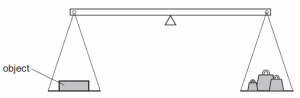Weights in the right-hand pan Effect 0.1 N, 0.1 N, 0.05 N, 0.02 N balance tips down slightly on the left-hand side 0.2 N, 0.1 N, 0.01 N balance tips down slightly on the right-hand side

What is the best estimate of the weight of the object?

2.The diagram shows a force being applied to a lever to lift a heavy weight.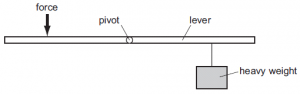Which change would enable the heavy weight to be lifted with a smaller force?

3.The diagram shows a uniform half-metre rule balanced at its mid-point.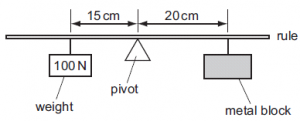What is the weight of the metal block?

4.A flat lamina is freely suspended from point P. The weight of the lamina is 2.0 N and the centre of mass is at C.

PC = 0.50 m

PQ = 0.40 m

QC = 0.30 m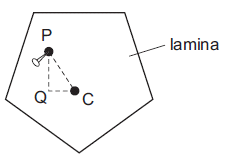The lamina is displaced to the position shown.

What is the moment that will cause the lamina to swing?

5.A piece of uniform card is suspended freely from a horizontal pin. At which of the points shown is its centre of gravity?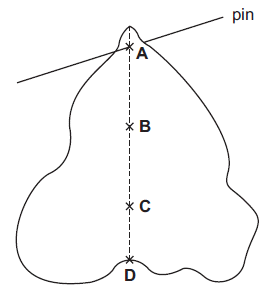6.A uniform metre rule is balanced by a 4 N weight as shown in the diagram.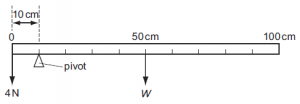What is the weight W of the metre rule?

 7 Which statement is true for a body in stable equilibrium?

 8 Which statement is true for a body in unstable equilibrium?

 9 How can the stability of an object be increased?

 10 If the total clockwise moment around a pivot is 10Nm and the total moment about a pivot is 0, what is the size of the anticlockwise moment?

Question 1 of 10